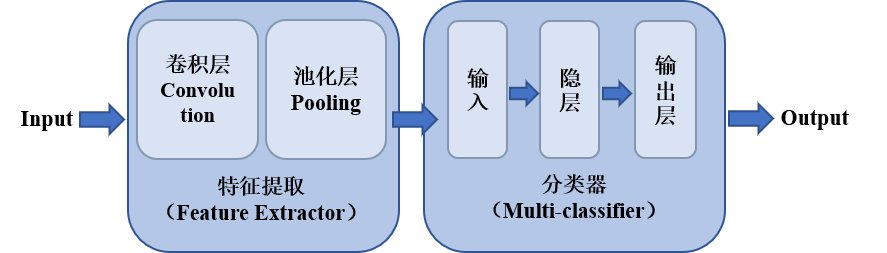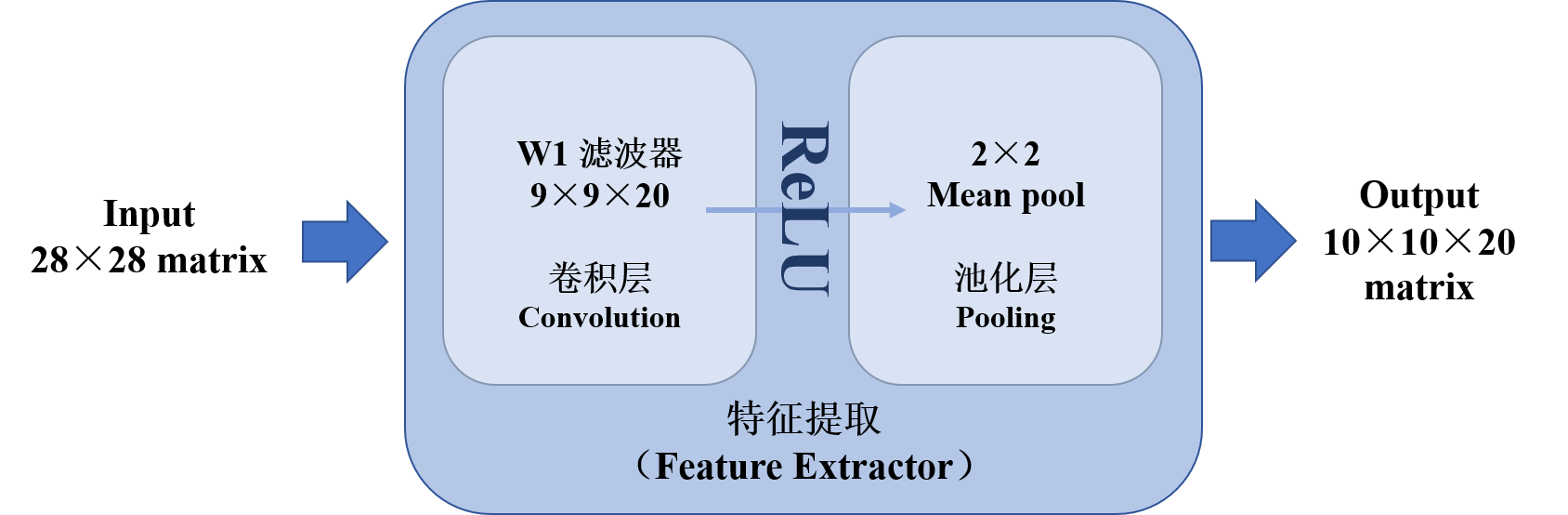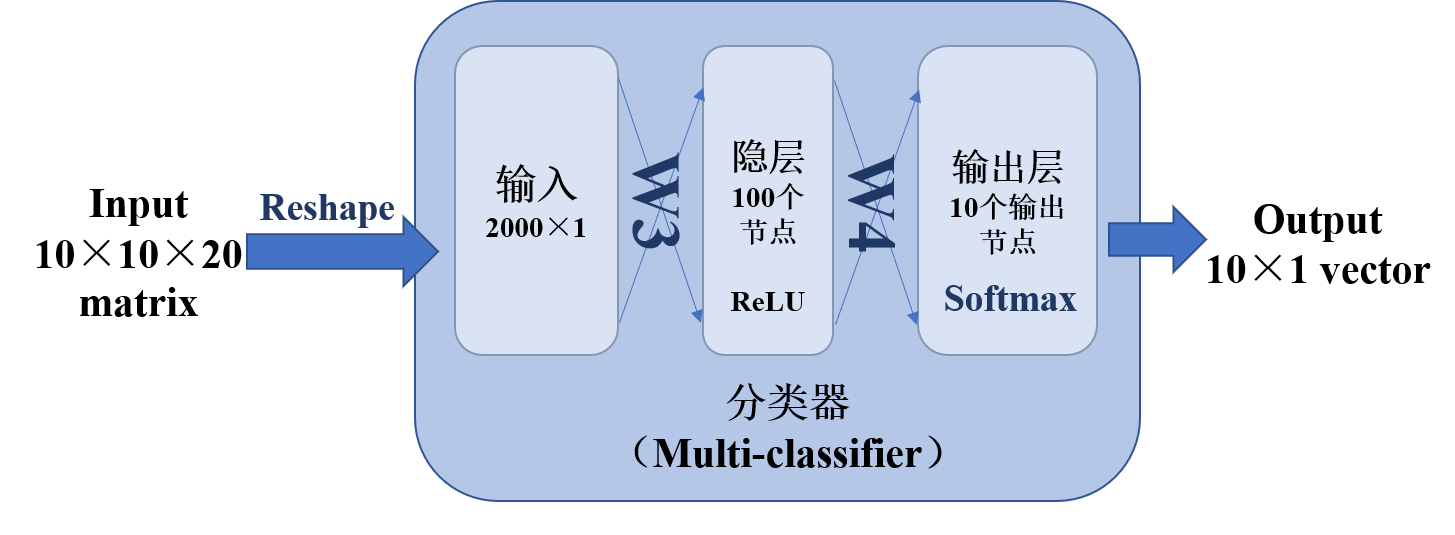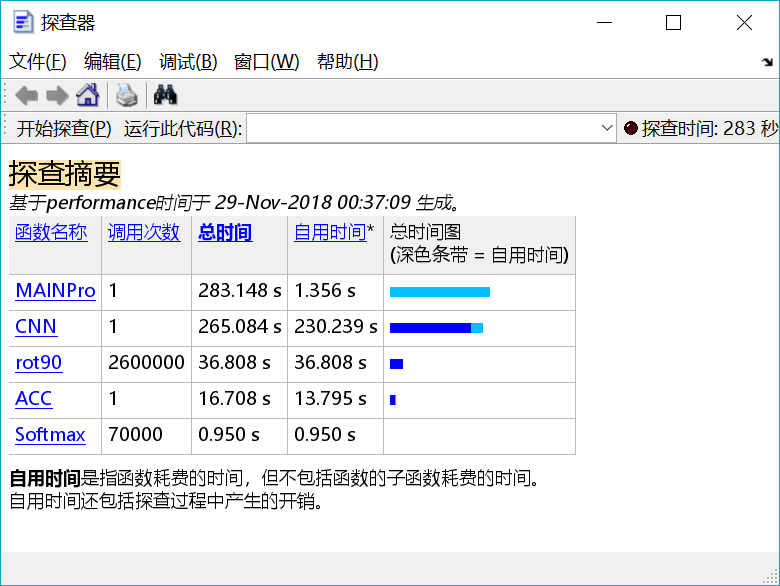2015-10-28 14:17:20 baimafujinji 阅读数 11370
• ###### 机器学习入门30天实战

购买课程后，可扫码进入学习群，获取唐宇迪老师答疑 系列课程包含Python机器学习库，机器学习经典算法原理推导，基于真实数据集案例实战3大模块。从入门开始进行机器学习原理推导，以通俗易懂为基础形象解读晦涩难懂的机器学习算法工作原理，案例实战中使用Python工具库从数据预处理开始一步步完成整个建模工作！具体内容涉及Python必备机器学习库、线性回归算法原理推导、Python实现逻辑回归与梯度下降、案例实战，信用卡欺诈检测、决策树与集成算法、支持向量机原理推导、SVM实例与贝叶斯算法、机器学习常规套路与Xgboost算法、神经网络。

7609 人正在学习 去看看 唐宇迪

# 图像处理中的数学原理详解（Part1 总纲）

http://blog.csdn.net/baimafujinji/article/details/48467225

1.3.3 方向导数与梯度2019-07-21 17:07:09 weixin_43348604 阅读数 45
• ###### 机器学习入门30天实战

购买课程后，可扫码进入学习群，获取唐宇迪老师答疑 系列课程包含Python机器学习库，机器学习经典算法原理推导，基于真实数据集案例实战3大模块。从入门开始进行机器学习原理推导，以通俗易懂为基础形象解读晦涩难懂的机器学习算法工作原理，案例实战中使用Python工具库从数据预处理开始一步步完成整个建模工作！具体内容涉及Python必备机器学习库、线性回归算法原理推导、Python实现逻辑回归与梯度下降、案例实战，信用卡欺诈检测、决策树与集成算法、支持向量机原理推导、SVM实例与贝叶斯算法、机器学习常规套路与Xgboost算法、神经网络。

7609 人正在学习 去看看 唐宇迪

def n4itk_norm(path,output_fn, n_dims=3, n_iters='[20,20,10,5]'):
'''
INPUT:  (1) filepath 'path': path to mha T1 or T1c file
(2) directory 'parent_dir': parent directory to mha file
OUTPUT: writes n4itk normalized image to parent_dir under orig_filename_n.mha
'''
# self.output_fn = output_fn
# output_fn = '/media/ycs/f_n4.png'
# run n4_bias_correction.py path n_dim n_iters output_fn
subprocess.call('python N4BiasCorrection.py ' + path + ' ' + str(n_dims) + ' ' + n_iters + ' ' + output_fn, shell = True)


N4BiasCorrection.py 的主程序如下：

from nipype.interfaces.ants import N4BiasFieldCorrection
import sys
import os
import ast

if len(sys.argv) < 2:
print("INPUT from ipython: run n4_bias_correction input_image dimension n_iterations(optional, form:[n_1,n_2,n_3,n_4]) output_image(optional)")
sys.exit(1)

# if output_image is given
if len(sys.argv) > 3:
n4 = N4BiasFieldCorrection(output_image=sys.argv)
else:
n4 = N4BiasFieldCorrection()

# dimension of input image, input image
n4.inputs.dimension = int(sys.argv)
n4.inputs.input_image = sys.argv

# if n_dinesions arg given
if len(sys.argv) > 2:
n4.inputs.n_iterations = ast.literal_eval(sys.argv)

n4.run()


2017-03-22 12:54:44 u014274339 阅读数 1195
• ###### 机器学习入门30天实战

购买课程后，可扫码进入学习群，获取唐宇迪老师答疑 系列课程包含Python机器学习库，机器学习经典算法原理推导，基于真实数据集案例实战3大模块。从入门开始进行机器学习原理推导，以通俗易懂为基础形象解读晦涩难懂的机器学习算法工作原理，案例实战中使用Python工具库从数据预处理开始一步步完成整个建模工作！具体内容涉及Python必备机器学习库、线性回归算法原理推导、Python实现逻辑回归与梯度下降、案例实战，信用卡欺诈检测、决策树与集成算法、支持向量机原理推导、SVM实例与贝叶斯算法、机器学习常规套路与Xgboost算法、神经网络。

7609 人正在学习 去看看 唐宇迪

## 图像处理的一些相关知识

### logistic transform

#### IQA算法中使用的扩展的logistic function

• 公式1
f(x)=τ1τ21+exp(xτ3τ4)+τ2

τ1,τ2,τ3,τ4使DMOSMOSMSE$\tau_1,\tau_2,\tau_3,\tau_4 是使得预测值和DMOS、MOS值的MSE最小的参数$
• 公式2
f(x)=β1(1211+exp(β2(xβ3)))+β4x+β5

β1,β2,β3,β4,β5使DMOSMOSMSE$\beta_1,\beta_2,\beta_3,\beta_4,\beta_5 是使得预测值和DMOS、MOS值的MSE最小的参数$
• matlab realization

### 梯度下降、最小二乘法

##### 最小二乘法和梯度下降法的关系
###### 相同
1. 本质相同：两种方法都是在给定已知数据（independent & dependent variables）的前提下对dependent variables算出出一个一般性的估值函数。然后对给定新数据的dependent variables进行估算。
2. 目标相同：都是在已知数据的框架内，使得估算值与实际值的总平方差尽量更小（事实上未必一定要使用平方），估算值与实际值的总平方差的公式为：
##### 不同
1. 实现方法和结果不同：最小二乘法是直接对求导找出全局最小，是非迭代法。而梯度下降法是一种迭代法，先给定一个，然后向下降最快的方向调整，在若干次迭代之后找到局部最小。梯度下降法的缺点是到最小点的时候收敛速度变慢，并且对初始点的选择极为敏感，其改进大多是在这两方面下功夫。

#### 性能评估

##### 皮尔森相关系数（Pearson correlation coefficient）
• 统计相关系数(1)——Pearson(皮尔逊)相关系数及MATLAB实现
• X、Y的皮尔森相关系数的含义
1. 当相关系数为0时，X和Y两变量无关系。
2. 当X的值增大（减小），Y值增大（减小），两个变量为正相关，相关系数在0.00与1.00之间。
3. 当X的值增大（减小），Y值减小（增大），两个变量为负相关，相关系数在-1.00与0.00之间。
• 公式
• 试用范围
• matlab 实现
1. matlab函数 corr()
2. 自写函数

#### 广义高斯概率分布

##### 基本概念

fX(x:μ,σ,γ)=ae[b|xμ|]γ

μ,σ2,γγ=2γ=1$\mu,\sigma^2,\gamma 分别是期望，方差和形状系数，\gamma=2时，高斯分布，\gamma=1时，拉普拉斯分布$##### 参数拟合与估计### 箱状图

#### 举例• 这组数据显示出：
• 最小值(minimum)=5
• 下四分位数(Q1)=7
• 中位数(Med)=8.5
• 上四分位数(Q3)=9
• 最大值(maximum )=10
• 平均值=8
• 最大值与最小值产生于这个区间。区间外的值被视为outlier显示在图上.
• mild outlier = 3.5
• extreme outlier = 0.5

### 离散余弦变换 DCT

#### 二维DCT变换#### 二维DCT反变换#### 分块DCT变换

$\quad$ 在实际的图像处理中，DCT变换的复杂度其实是比较高的，所以通常的做法是，将图像进行分块，然后在每一块中对图像进行DCT变换和反变换，在合并分块，从而提升变换的效率。具体的分块过程中，随着子块的变大，算法复杂度急速上升，但是采用较大的分块会明显减少图像分块效应，所以，这里面需要做一个折中，在通常使用时，大都采用的是8*8的分块。### 离散小波变换 DWT

#### 一维#### 二维#### 实际范例2018-11-29 01:20:48 ercong_kang 阅读数 810
• ###### 机器学习入门30天实战

购买课程后，可扫码进入学习群，获取唐宇迪老师答疑 系列课程包含Python机器学习库，机器学习经典算法原理推导，基于真实数据集案例实战3大模块。从入门开始进行机器学习原理推导，以通俗易懂为基础形象解读晦涩难懂的机器学习算法工作原理，案例实战中使用Python工具库从数据预处理开始一步步完成整个建模工作！具体内容涉及Python必备机器学习库、线性回归算法原理推导、Python实现逻辑回归与梯度下降、案例实战，信用卡欺诈检测、决策树与集成算法、支持向量机原理推导、SVM实例与贝叶斯算法、机器学习常规套路与Xgboost算法、神经网络。

7609 人正在学习 去看看 唐宇迪

# 关于图像处理——运用CNN实现数字手写体识别的调研

FROM 百度百科-卷积神经网络

## 正文### 数据处理

• 训练数据用0-1编码，以便进行矩阵运算。本例中单个输入样本为28×28矩阵。
• 输出结果采用单热编码（e.g. ‘1’=[1 0 0 0 0 0 0 0 0 0]，仅用于分类区分），以便于网络设计与统计。

### 特征提取（Feature Extractor）• #### 卷积层设计

卷积层采用20个9×9的滤波器，滤波结果选取valid部分。其后运用ReLU作为激活函数，传入池化层。
• #### 池化层设计

池化层输入为20个20×20的矩阵。本例池化层采用2×2平均池化，即每四个输出为一个单元，取这四个数的均值为新的输出。最后的池化结果为20个10×10的矩阵。

### 分类器（Multi-classifier）• #### 输入层设计

1. 从特征提取网络传入的单组样本为20个10×10的矩阵（因为有20个滤波器，故而每一个输入样本有20组输出），为方便之后的分类训练，我们将其转化为一个2000×1的向量；
2. 设置第一层权重矩阵大小为100×2000；
3. 运用ReLU作为第一层的激活函数。
• #### 隐层设计

1. 设置隐层权重矩阵大小为10×100；
2. 设置激活函数为ReLU。
• #### 输出层设计

1. 设置输出层激活函数为Softmax（用于多分类问题的激活函数）；
2. 最终输出结果为10×1的向量，最大值对应表征为1，其余值表征为0。

### 后向传播的实现（Backward）（说明：J为代价函数；w为权重矩阵；e为误差；x为前层输出）

## 算法实现

### 主程序部分

% this is my main program for MNIST
% CNN and BP are used to build whole structure
% this is a basic one
% name: 余尔聪
% date: 2018-11-28

% import TRAINING and TESTING Data

% initiation of variables
X = X_Train; % training data-input
D = D_Train; % training data-output
W1 = randn(9,9,20); % 9×9 Convolution Matrix
W3 = (2*rand(100,2000)-1)/20; % 1st layer Weight Matrix
W4 = (2*rand(10,100)-1)/10; % 2nd layer Weight Matrix

% Training for 1 time
[W1,W3,W4] = CNN(X,D,W1,W3,W4);

% Show Accuracy
acc = ACC(D_Test,X_Test,W1,W3,W4);
fprintf('Accuracy is %f\n', acc);


### CNN-训练部分

function [W1,W3,W4] = CNN(X,D,W1,W3,W4)
% this is the training function
% comprised of Feature Extractor and Multi-classifier
% inputs include Training input and output, W1, W2, W3
% aims to find proper W1, W2, W3 as output

dW1 = zeros(9,9,20); % preallocating for being faster
alpha = 0.01; % step length
for k = 1:60000 % one epoch for one sample
x = X(:,:,k); % initiate one epoch input
d = D(:,k); % initiate one epoch output


#### 前向传播（Forward）

• ##### 特征提取（Feature Extractor）
        % Forward Algorithm

% Feature Extractor
% comprised of Convolution and Pooling
for m = 1:20
V1(:,:,m) = conv2(x,rot90(W1(:,:,m),2),'valid'); % Convolution Algorithm
end
Y1 = max(0,V1); % Activation Function-ReLU
Y2 = (Y1(1:2:end,1:2:end,:)+Y1(2:2:end,1:2:end,:)+Y1(1:2:end,2:2:end,:)+Y1(2:2:end,2:2:end,:))/4;
% Pooling by 2×2 Mean

• ##### 分类器（Multi-classifier）
        % Multi-classifier
y2 = reshape(Y2,[],1); % reshape Y2 into 2000×1 vector
v3 = W3*y2; % 1st layer calculation
y3 = max(0,v3); % ReLU
v = W4*y3; % 2nd layer calculation
y = Softmax(v); % Softmax-multiclassification


#### 后向传播（Backward）

• ##### 分类器（Multi-classifier）
        % BP Algorithm
% Multi-classifier
e = d-y; % error of output
delta = e; % cross_entropy+Softmax
e3 = W4'*delta; % error of 2nd layer
delta3 = (v3>0).*e3; % ReLU
e2 = W3'*delta3; % error of 1st layer

dW4 = alpha*delta*y3'; % change in W4
dW3 = alpha*delta3*y2'; % change in W3

W3 = dW3+W3; % update W3
W4 = dW4+W4; % update W4

• ##### 特征提取（Feature Extractor）
        % Feature Extractor
E2 = reshape(e2,size(Y2)); % reshape e2 into 10×10×20 matrix
E1 = zeros(size(Y1));E2_4 = E2/4; % backward pooling
E1(1:2:end,1:2:end,:) = E2_4;
E1(1:2:end,2:2:end,:) = E2_4;
E1(2:2:end,1:2:end,:) = E2_4;
E1(2:2:end,2:2:end,:) = E2_4;
delta1 = (V1>0).*E1; % ReLU
for m = 1:20
dW1(:,:,m) = alpha*conv2(x,rot90(delta1(:,:,m),2),'valid');
% change in W1
end
W1 = W1+dW1; % update W1


### 结果检测

function acc = ACC(D_Test,X_Test,W1,W3,W4)
% This is Used to Show Accuracy
N = length(D_Test);
d_comp = zeros(1,N);
for k = 1:N
x = X_Test(:,:,k); % initiate one epoch input
% Feature Extractor
% comprised of Convolution and Pooling
for m = 1:20
V1(:,:,m) = conv2(x,rot90(W1(:,:,m),2),'valid'); % Convolution Algorithm
end
Y1 = max(0,V1); % Activation Function-ReLU
Y2 = (Y1(1:2:end,1:2:end,:)+Y1(2:2:end,1:2:end,:)+Y1(1:2:end,2:2:end,:)+Y1(2:2:end,2:2:end,:))/4;
% Pooling by 2×2 Average

% Multi-classifier
y2 = reshape(Y2,[],1); % reshape Y2 into 2000×1 vector
v3 = W3*y2; % 1st layer calculation
y3 = max(0,v3); % ReLU
v = W4*y3; % 2nd layer calculation
y = Softmax(v); % Softmax-multiclassification

% Statistics Accuracy
[~, i] = max(y);
d_comp(k) = i;
end
[~, d_true] = max(D_Test); % real output
correctMsk = (d_comp == d_true); % counting
acc = sum(correctMsk)/N; % rate calculation
end



## 结语

### 结果分析MAINPro
Accuracy is 0.975800

### 网络优化

2012-04-09 16:30:33 icemanyandy 阅读数 1208
• ###### 机器学习入门30天实战

购买课程后，可扫码进入学习群，获取唐宇迪老师答疑 系列课程包含Python机器学习库，机器学习经典算法原理推导，基于真实数据集案例实战3大模块。从入门开始进行机器学习原理推导，以通俗易懂为基础形象解读晦涩难懂的机器学习算法工作原理，案例实战中使用Python工具库从数据预处理开始一步步完成整个建模工作！具体内容涉及Python必备机器学习库、线性回归算法原理推导、Python实现逻辑回归与梯度下降、案例实战，信用卡欺诈检测、决策树与集成算法、支持向量机原理推导、SVM实例与贝叶斯算法、机器学习常规套路与Xgboost算法、神经网络。

7609 人正在学习 去看看 唐宇迪